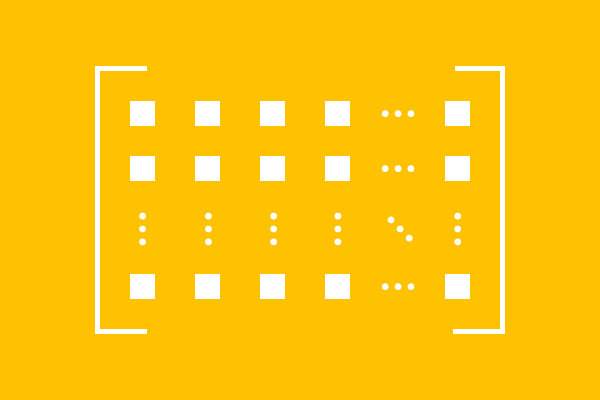# Rectangular Matrix

A matrix whose number of rows does not equal to the number of columns, is called a rectangular matrix.

## Introduction

Rectangular matrix is one type of matrix. In this matrix, the elements are arranged in rows and columns. The arrangement of elements in the matrix represents a rectangle shape. Hence, it is called a rectangular matrix.For example, we have some elements, in which $n$ elements are arranged in each row. Thus, all elements are arranged in $m$ rows. Therefore, the elements are arranged in $m$ rows and $n$ columns.

Now, we have a matrix of the order $m \times n$ and it can be expressed in mathematical form as follows.

$M$ $\,=\,$
${\begin{bmatrix} e_{11} & e_{12} & e_{13} & \cdots & e_{1n}\\ e_{21} & e_{22} & e_{23} & \cdots & e_{2n}\\ e_{31} & e_{32} & e_{33} & \cdots & e_{3n}\\ \vdots & \vdots & \vdots & \ddots & \vdots \\ e_{m1} & e_{m2} & e_{m3} & \cdots & e_{mn} \end{bmatrix}}_m \times n}$

Geometrically, the rectangle shape in a matrix is possible if the number of rows is different to the number of columns. It means $m \ne n$.

Therefore, there are two possible cases in forming a rectangular matrix, one is the number of rows is greater than the number of columns ($m > n$) and the other is the number of rows is less than the number of columns ($m < n$).

Now, let’s learn the concept of rectangular arrangement of elements with some understandable examples.

### Rows less than Columns

$A$ is a matrix of the order $3 \times 4$.

$A$ $\,=\,$
$\begin{bmatrix} 5 & -1 & 4 & 9\\ -7 & 1 & 3 & 2\\ 8 & 5 & 0 & -6 \end{bmatrix}$

In the matrix $A$, the elements are arranged in $3$ rows and $4$ columns. In this matrix, the number of rows is not equal to the number of columns $(3 \ne 4)$ but the number of rows is less than the number of columns $(3 < 4)$. It is the main reason for the formation of rectangle shape in this matrix. Hence, the matrix $A$ is called a rectangular matrix.

### Rows greater than Columns

$B$ is another matrix and the order of this matrix is $5 \times 2$.

$B$ $\,=\,$
$\begin{bmatrix} 2 & 6\\ 5 & 2\\ 9 & 4\\ 6 & 2\\ 7 & -6 \end{bmatrix}$

In the matrix $B$, the elements are arranged in $5$ rows and $2$ columns. So, the number of rows is not equal to the number of columns $(5 \ne 2)$ in this matrix but the number of rows is greater than the number of columns $(5 > 2)$. It is the actual reason for the formation of rectangle shape in this matrix. Therefore, the matrix $B$ is also called a rectangular matrix.

Latest Math Topics
Jun 26, 2023
Jun 23, 2023

Latest Math Problems
Jul 01, 2023
Jun 25, 2023
###### Math Questions

The math problems with solutions to learn how to solve a problem.

Learn solutions

Practice now

###### Math Videos

The math videos tutorials with visual graphics to learn every concept.

Watch now

###### Subscribe us

Get the latest math updates from the Math Doubts by subscribing us.